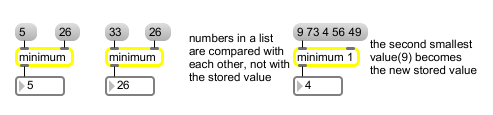# minimum

Output the smallest in a list of numbers

## Description

minimum watches an input stream for any number which is smaller than its most recently set minimum, sets that new number as the minimum, and outputs it.

## Arguments

Name Type Opt Description
initial-comparison-value int or float opt Sets an initial value to be compared with numbers received in the left inlet. If the argument contains a decimal point, all numbers are compared as floats, and the output is a float. If there is no argument, the initial value is 0.

## Messages

 bang In left inlet: Sends the most recent output out the outlet again. int input [int] In left inlet: If the number is less than the value currently stored in minimum, it is sent out the outlet. Otherwise, the stored value is sent out. (inlet1) comparison-value [int] In right inlet: The number is stored for comparison with subsequent numbers received in the left inlet. float input [float] In left inlet: Converted to int, unless there is a float argument, in which case all numbers are compared as floats. (inlet1) comparison-value [float] In right inlet: Converted to int, unless there is a float argument, in which case all numbers are compared as floats. list input [list] In left inlet: The numbers in the list are all compared to each other, and the smallest value is sent out the outlet. The value stored in minimum is replaced by the next smallest value in the list. The minimum object accepts lists of up to 256 elements.

## Output

float: Floats are output only if there is an argument with a decimal point.
int: The number received in the left inlet is compared with the value currently held by minimum (or numbers received as a list are compared with each other), and the smallest of the numbers is sent out the outlet.

## ExamplesThe output is the lesser of two numbers or the smallest in a list of numbers